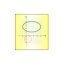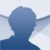# Math Studio

3.5

## Math Studio is focused on the creation of mathematical graphics. Download Math Studio and obtain graphical representations of any mathematical function

Mathematics is an exact science with a great degree of logic and abstraction. Due to this, it is sometimes very difficult to imagine mathematical concepts as something tangible. And that is why one of the best ways to understand mathematics is by means of graphical representations. For this purpose, we have a complete tool: Math Studio.

### Start to understand mathematics visually

Math Studio is a utility that allows the generation of graphs from mathematical functions, algebraic expressions, etc. It's an application with great didactic value, that can be of great use to both teachers and students.The application allows us to obtain graphics of explicit, implicit, parametric, piecewise, and inequality form functions, analytic geometry, numerical sequences, etc. It allows Cartesian and polar coordinates. Furthermore, it provides other functions such as the possibility to create and save animations, use an expression calculator, rotate and move the graphics that are on the screen or save them to a file.